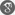##### Cite as:
Nsisong Ekong, Imoh Moffat, Anthony Usoro, Iseh Matthew, A Comparative Study of the Impact of Dummy Variables on Regression Coefficients and Canonical Correlation Indices: An Empirical Perspective, Int. J. Anal. Appl., 19 (4) (2021), 576-586.

#### Abstract

In this paper, the impact of dummy variables on regression coefficients and canonical correlation indices from an empirical perspective is investigated. To do this, a regression analysis of Crude Oil Prices on US dollars - Naira Exchange Rates is performed, and the extent of the significance of the relationship is noted. Secondly, dummy variables (coded with respect to various economic regimes of interest) is introduced into the regression of the two variables and the impact of such introduction is also noted. And also, a canonical correlation analysis (CCA) of Inflation rate, the dummy variables and Crude Oil Prices and the dummy variables is conducted. Finally, we compare the significant role of the introduction of the dummy variables on the coefficients of the regression and the canonical correlation indices. The results showed that the introduction of dummy variables impact more on the canonical correlation indices than it does on the regression coefficients.

#### References

1. J.G. Lutz, T.L. Eckert, The relationship between canonical correlation analysis and multivariate multiple regression, Educ. Psychol. Measure. 54 (1994), 666–675.2. N.R. Draper, H. Smith, Applied regression analysis. 2nd ed. Wiley, New York, (1981).3. B.S. Everitt, Multiple Regression and Canonical Correlation, in: An R and S-PLUS® Companion to Multivariate Analysis, Springer London, London, 2005: pp. 157–170.4. T. Foucart, Paper on multiple linear regression on canonical correlation variable.Biometrical J. 41 (1998), 559-572.5. L.K. George, A comparison of canonical correlation and multiple regression in the analysis of change. In: Proceedings of the 1975 Joint Statistical Association, (1975), 262-269.6. S.M. Kakade, D.P. Foster, Multi-view Regression via Canonical Correlation Analysis. In: International Conference on Computational Learning Theory, (2007), 82-96.7. L. Sun, S. Ji, J. Ye, Least Squares Formulations for Canonical Correlation Analysis. In: International Conference on Machine Learning, (2008), 1024-1031.8. R.E. Walpole, R.H. Myers,Probability and Statistics for Engineers and Scientists. 4th ed. Macmillan Publishing Company, New York, (1989).